# Mixed fractions calculator

The calculator performs basic and advanced operations with mixed numbers, fractions, integers, decimals. Mixed fractions are also called mixed numbers. A mixed fraction is a whole number and a proper fraction combined, i.e. one and three-quarters. The calculator evaluates the expression or solves the equation with step-by-step calculation progress information. Solve problems with two or more mixed numbers fractions in one expression.

## Result:

### 1/4 - 2/3 = -5/12 ≅ -0.4166667

Spelled result in words is minus five twelfths.

### Calculation steps

1. Subtract: 1/4 - 2/3 = 1 · 3/4 · 3 - 2 · 4/3 · 4 = 3/12 - 8/12 = 3 - 8/12 = -5/12
For adding, subtracting, and comparing fractions, it is suitable to adjust both fractions to a common (equal, identical) denominator. The common denominator you can calculate as the least common multiple of both denominators - LCM(4, 3) = 12. In practice, it is enough to find the common denominator (not necessarily the lowest) by multiplying the denominators: 4 × 3 = 12. In the following intermediate step, it cannot further simplify the fraction result by canceling.
In other words - one quarter minus two thirds = minus five twelfths.

#### Examples:

sum of two mixed numbers: 1 3/4 + 2 3/8
addition of three mixed numbers: 1 3/8 + 6 11/13 + 5 7/8
addition of two mixed numbers: 2 1/2 + 4 2/3
subtracting two mixed numbers: 7 1/2 - 5 3/4
multiplication of mixed numbers: 3 3/4 * 2 2/5
comparing mixed numbers: 3 1/4 2 1/3
changing improper fraction to mixed number: 9/4
What is 3/4 as a mixed number: 3/4
subtracting mixed number and fraction: 1 3/5 - 5/6
sum mixed number and an improper fraction: 1 3/5 + 11/5

## Mixed fractions in word problems:

• If you 5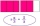If you subtract N from 8 2/5 the difference is 3 4/3. what is N?
• Peter's calculation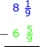Peter wrote the following: 7 1/4 - 3 3/4 = 4 2/4 = 4 1/2 . Is Peter’s calculation correct? Using words (math vocabulary) and numbers explain why he is correct or incorrect.
• Subtract mixedFind the difference. 5 and two-thirds minus 2 and one-half equals what number? A. three and one-sixth B. three and two-thirds C. three and one-half D. three and five-sixths
• How much 3How much more is 1⅝ than 4⅚?
• Zailene 2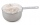Zailene has 8 2/3 cup of flour. She used 5 1/2 cups of flour. How many cups of flour was left to her?
• SubtractSubtract two mixed numbers: 3 1/2 -1 3/4
• Sum of 20Sum of the two numbers is 15 1/6. If one of the numbers is 2 2/3, find the other number.
• Equation with mixed 2A number, X, is subtracted from 8 1/4. The result is 12 3/5. What is the value of X?
• Difference mixed fractionsWhat is the difference between 4 2/3 and 3 1/6?
• Bike pathBill was riding his bike on a bike path that was 5 1/4 miles long. He rode his bike awhile and stopped for s drink or water. Then he rode 2 7/10 miles and was at the end of the path. How far had Bill ridden before he stopped for water?
• TyronTyron attended his online class 2 hours a day. He already attend 1 3/4 hours, how many more hours will Tyron attend his class?
• Denissa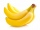Denissa has 18 9/10 kilos of bananas to sell if she was able to sell 15 ⅗. How many kilos of bananas were left?
• Light-years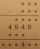Planet X is 10 light-years away from Earth. Planet Y is 8 1/2 light-years away from Earth. How much farther away is Planet X?
• Sum of mixedThe sum of two mixed numbers is 15½. One of the number is 5 ¾, what is the other?
• Sum of 18Sum of two fractions is 4 3/7. If one of the fractions is 2 1/5 find the other one .
• Cups of punchCyka made 6 19/20 cups of punch punch at two different types of juice in it. If the punch had 4 1/5 cups of one type of juice how many cups of the other type of juice did it have?
• GavinGavin had 3 gallons of orange juice ready to serve at the breakfast party. His family drank 2 ⅛ gallons. How much is left? Show your work with a numberline, area model or grid.